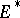# Contravariant vector

(diff) ← Older revision | Latest revision (diff) | Newer revision → (diff)
Jump to: navigation, search

The name of an element of a vector spacein the case when the dual (or conjugate) space (cf. Adjoint space)is considered along with. The elements ofare then called covariant vectors (cf. Covariant vector).

#### Comments

The word "vector" or the phrase "contravariant vector" is also used to denote a vector field. Cf. also Tensor on a vector space; Contravariant tensor; Tensor bundle.

How to Cite This Entry:
Contravariant vector. Encyclopedia of Mathematics. URL: http://www.encyclopediaofmath.org/index.php?title=Contravariant_vector&oldid=14637
This article was adapted from an original article by M.I. Voitsekhovskii (originator), which appeared in Encyclopedia of Mathematics - ISBN 1402006098. See original article